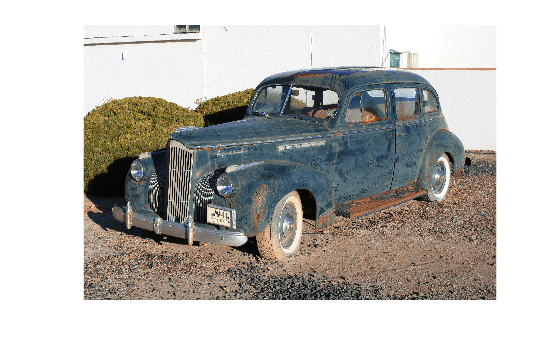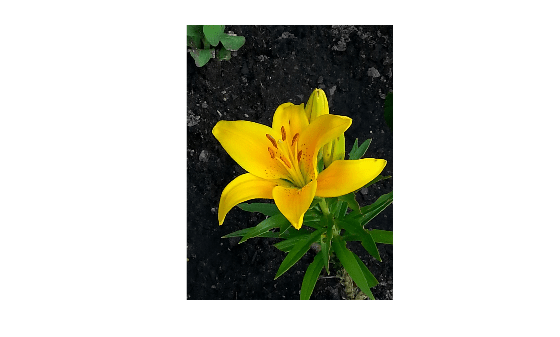# fitniqe

Fit custom model for NIQE image quality score

## Syntax

``model = fitniqe(imds)``
``model = fitniqe(imds,Name,Value)``

## Description

example

````model = fitniqe(imds)` creates a Naturalness Image Quality Evaluator (NIQE) model from reference image datastore `imds`.```

example

````model = fitniqe(imds,Name,Value)` creates a NIQE model using additional parameters to control the model calculation.```

## Examples

collapse all

Load a set of natural images into an image datastore. These images are shipped in Image Processing Toolbox™ in a directory named 'imdata'.

```setDir = fullfile(toolboxdir('images'),'imdata'); imds = imageDatastore(setDir,'FileExtensions',{'.jpg'});```

Train a custom NIQE model using the image datastore.

`model = fitniqe(imds);`
```Extracting features from 38 images. . Completed 3 of 38 images. Time: Calculating... ... Completed 12 of 38 images. Time: 00:26 of 01:01 ... Completed 18 of 38 images. Time: 00:36 of 01:06 .... Done. ```

Read an image of a natural scene. Display the image.

```I = imread('car1.jpg'); imshow(I)```Calculate the NIQE score for the image using the custom model. Display the score.

```niqeI = niqe(I,model); fprintf('NIQE score for the image is %0.4f.\n',niqeI)```
```NIQE score for the image is 1.8730. ```

Load a set of natural images into an image datastore. These images are shipped in Image Processing Toolbox™ in a directory named 'imdata'.

```setDir = fullfile(toolboxdir('images'),'imdata'); imds = imageDatastore(setDir,'FileExtensions',{'.jpg'});```

Create the custom model of NSS features using the image datastore. Specify a block size and use the default sharpness threshold.

`model = fitniqe(imds,'BlockSize',[48 96])`
```Extracting features from 38 images. . Completed 3 of 38 images. Time: Calculating... .... Completed 14 of 38 images. Time: 00:23 of 00:50 ... Completed 24 of 38 images. Time: 00:33 of 00:49 .... Done. ```
```model = niqeModel with properties: Mean: [1.9291 0.5854 0.6735 0.0667 0.0517 0.0889 ... ] Covariance: [36x36 double] BlockSize: [48 96] SharpnessThreshold: 0 ```

Read a natural image into the workspace. Display the image.

```I = imread('yellowlily.jpg'); imshow(I)```Calculate the NIQE score for the image using the custom model. Display the score.

```niqeI = niqe(I,model); fprintf('NIQE score for the image is %0.4f.\n',niqeI)```
```NIQE score for the image is 2.9558. ```

## Input Arguments

collapse all

Reference image datastore, specified as an `ImageDatastore` object. The datastore must contain 2-D grayscale or 2-D RGB images of data type `single`, `double`, `int16`, `uint8`, or `uint16`.

### Name-Value Arguments

Specify optional comma-separated pairs of `Name,Value` arguments. `Name` is the argument name and `Value` is the corresponding value. `Name` must appear inside quotes. You can specify several name and value pair arguments in any order as `Name1,Value1,...,NameN,ValueN`.

Example: `model = fitniqe(imds,'BlockSize',[48 36])` fits a NIQE model using 48-by-36 pixel blocks.

Block size used to partition the images, specified as the comma-separated pair consisting of `'BlockSize'` and a 2-element row vector of positive even integers. Blocks are nonoverlapping. Natural scene statistics, which are calculated from the blocks, define the output `model`.

Data Types: `single` | `double` | `int8` | `int16` | `int32` | `uint8` | `uint16` | `uint32`

Sharpness threshold, specified as the comma-separated pair consisting of `'SharpnessThreshold'` and a numeric scalar in the range [0, 1]. The sharpness threshold, `s`, controls which image blocks are used to compute the model. `fitniqe` computes the model using all blocks that have sharpness more than `s` times the maximum sharpness among all blocks.

Data Types: `single` | `double`

## Output Arguments

collapse all

Custom model of image features, returned as a `niqeModel` object.

## Tips

• The custom dataset specified in the image datastore `imds` should consist of images that are perceptually pristine to human subjects. However, the definition of pristine depends on the application. For example, a pristine set of microscopy images has a different set of quality criteria than images of buildings or outdoor scenes. When training a custom NIQE model, use images with varied image content and with potentially different sets of quality criteria.

 Mittal, A., R. Soundararajan, and A. C. Bovik. "Making a Completely Blind Image Quality Analyzer." IEEE Signal Processing Letters. Vol. 22, Number 3, March 2013, pp. 209–212.